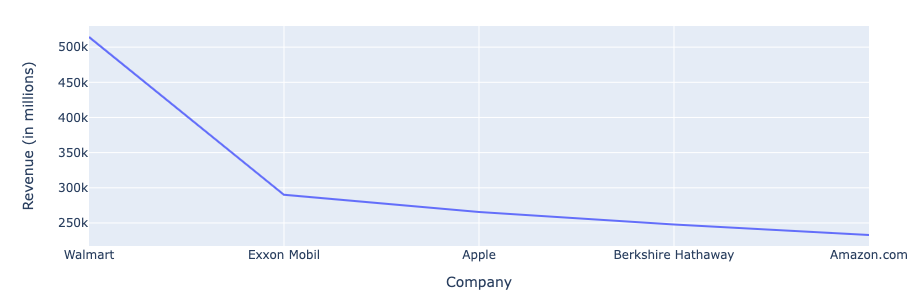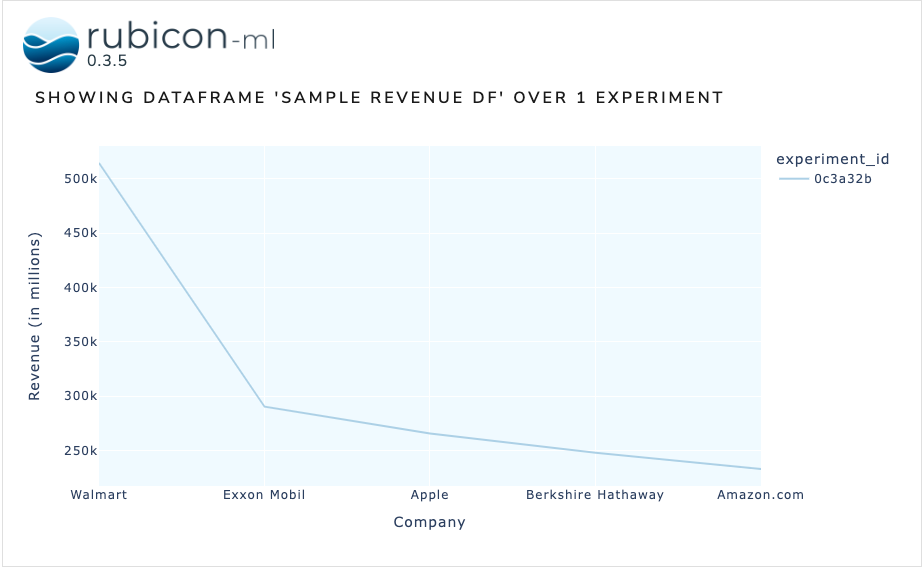# Visualize Logged Dataframes#

rubicon_ml makes it easy to log dataframes and comes with default visualization support, using Dash and Plotly under the hood. Specifically, rubicon_ml leverages Plotly express to provide many robust visualization options.

## Setup#

Let’s create a project and log a sample dataframe to it so we can visualize it.

:

import os

from rubicon_ml import Rubicon

rubicon = Rubicon(persistence="memory")
project = rubicon.get_or_create_project("Plotting Example")

project

:

<rubicon_ml.client.project.Project at 0x1629cf2e0>

:

import pandas as pd

df = pd.DataFrame.from_records(
[
["Walmart", 514405],
["Exxon Mobil", 290212],
["Apple", 265595],
["Berkshire Hathaway", 247837],
["Amazon.com", 232887]
],
columns=["Company", "Revenue (in millions)"]
)

experiment = project.log_experiment()
dataframe = experiment.log_dataframe(df, name="sample revenue df")

dataframe

:

<rubicon_ml.client.dataframe.Dataframe at 0x1629ce170>


## Visualizing Dataframes#

rubicon_ml.Dataframe.plot exposes plotting functionality to create simple plots like line, bar, or scatter plots and tables. There are even more complex plots available in plotly.express - any can be passed into rubicon_ml for visualization!

Here we’ll visualize the dataframe with the default line chart (px.line). Any keyword arguments will be passed directly to the underlying Plotly express function.

Note: If you’re viewing this notebook from the rubicon_ml docs, the plots and widget below are only images since we can’t run a Dash server on GitHub pages. Running locally will produce fully interactive plots!

:

revenue_line = dataframe.plot(x="Company",  y="Revenue (in millions)")

revenue_lineThis time we’ll provide px.scatter as the plotting_func argument to generate a scatter plot.

:

import plotly.express as px

revenue_scatter = dataframe.plot(
plotting_func=px.scatter,
x="Company",
y="Revenue (in millions)",
)

revenue_scatterDataframes can also be visualzied with the dataframe plot widget. For more info the dataframe plot, check out this example. For more info on rubicon_ml’s visualization widgets in general, check out the docs.

:

from rubicon_ml.viz import DataframePlot

DataframePlot(
experiments=[experiment],
dataframe_name="sample revenue df",
).show()

Dash is running on http://127.0.0.1:8050/